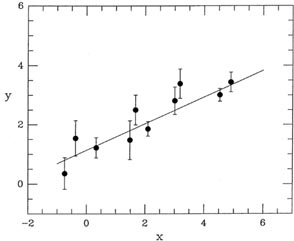### LINEAR LEAST SQUARES

One of the most common tasks in observational astronomy is to derive the "best" numerical relationship between observable quantities, where some or all of the data that you are analyzing contain measuring errors. The most widely used and best understood tool for carrying out this sort of analysis is the so-called "method of least-squares." This is a very powerful technique, and one which is useful in a wide variety of data-reduction problems. Let me begin to explain how least squares works by looking at a situation I'm sure you are all familiar with: you need to fit a straight line to two-dimensional data, where the data are subject to measuring errors in one of the dimensions (Fig. 1-1).Figure 1-1